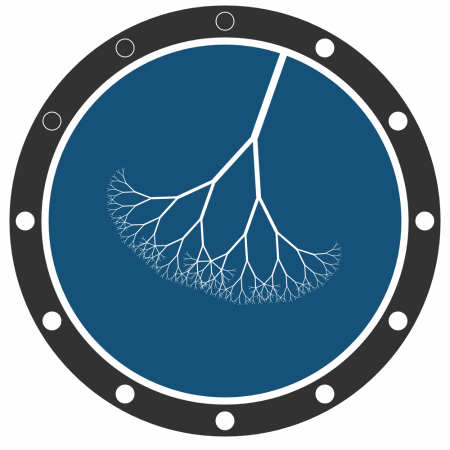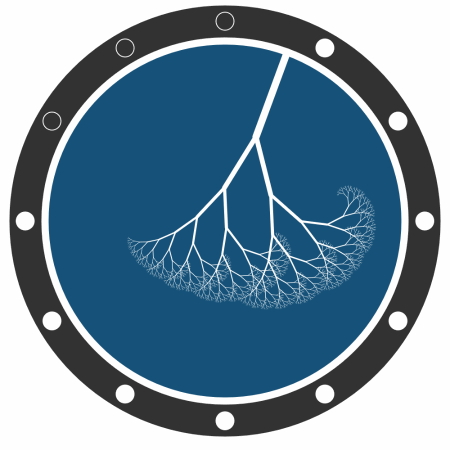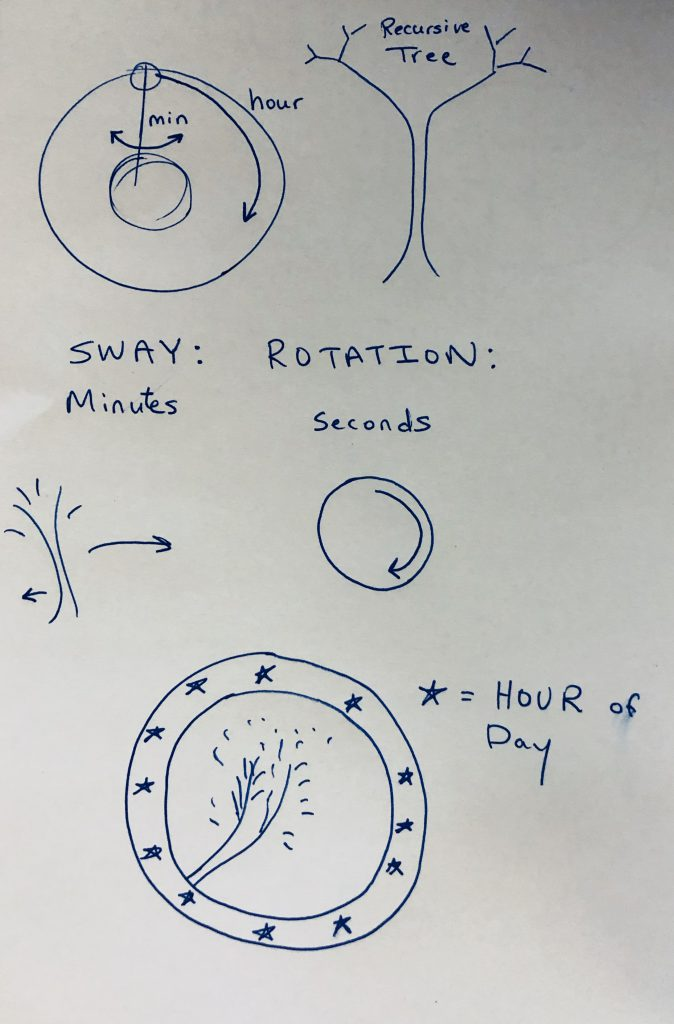# Zaport-clock

The principal element of the clock is the recursive tree. A second is represented by the leaves pulsing in and out. A minute is represented by the rotation of the tree around the perimeter of the clock face. An hour is represented by the left-right position of the tree, which resembles a sway. Finally, 12-hour cycle is represented by the circles in the border of the clock. This exercise taught me a lot about the translate function in p5js, which I used throughout. The clock is simply made and has a simple aesthetic. I was hoping to create a clock that resembled time in a smooth and uninterrupted way.

Here are some GIFs from the 9-o’clock hour (9:00, 9:15, 9:30, 9:45):Here’s the current time using the code with openProcessing:

Here are my sketches:Here is my code:

```//Zaport //Clock for 60-212 //2018   var xc var yc var xSide = 500 var ySide = xSide var bigRadius = 200   function setup() { createCanvas(xSide, ySide); }   function draw() {   //https://www.openprocessing.org/sketch/387974 //by Jacob Joaquin //translate(width / 2.0, height / 2.0); var date = new Date(), hours = abs(12-(date.getHours())), minutes = date.getMinutes(), seconds = date.getSeconds(), ms = date.getMilliseconds(), i, v, t;   // Minute hand t = (seconds + ms / 1000) * TAU / 60 - HALF_PI; v = p5.Vector.fromAngle(t); v.mult(bigRadius); xc = v.x yc = v.y   var smoothTime = (0.01*seconds)+minutes   background(255); smooth()   conditionalTime = abs(30-smoothTime) if (0 == hours%2) { angleRight = (conditionalTime/75) angleLeft =(60-conditionalTime)/75 }else{ angleLeft = (conditionalTime/75) angleRight =(60-conditionalTime)/75 } if (0 == seconds%2) { numberBranches = ms/50 }else { numberBranches = 10-(ms/50) }   fill(50) ellipse(width/2,height/2,bigRadius*2+80,bigRadius*2+80); stroke(255); strokeWeight(8) fill(21, 81, 120); ellipse(width/2,height/2,bigRadius*2,bigRadius*2);   push(); translate(width/2,height/2); translate(xc, yc); rotate(t - radians(90)); fill(255) branch(100,8); pop();   // Minute Markers fill(255); strokeWeight(1); for (i = 0; i &lt; 60; i++) { v = p5.Vector.fromAngle((i + 1) / 60.0 * TAU - HALF_PI); v.mult(bigRadius+22); if (i % 5 == 4) { push() if (i &lt; (hours*5)) { fill(255) } else { fill(50) } translate(width/2,height/2); ellipse(v.x, v.y, 20, 20); pop() } } } //Recurive tree code adapted from code by Mary Notari (marinotari) //https://alpha.editor.p5js.org/marynotari/sketches/HkAiz5Jxf function branch (len,strokeThickness) { strokeWeight(strokeThickness); line(0, 0, 0, -len); translate(0, -len); var fraction = 2/3; var weightFraction = 2/3 if(len &gt; numberBranches &amp;&amp; len &gt; 1) { push(); rotate(angleLeft); branch(len*fraction,strokeThickness*weightFraction); pop();   push(); rotate(-angleRight); branch(len*fraction,strokeThickness*weightFraction); pop(); }   }```